## C语言初学者，请教数组指针问题

#include<stdio.h>
int main(void)
{

char p = "Hello Word!";
char (*q)  = &p;
printf("%s \n",*q);
printf("\n");
int a = {1,2,3,4,5};
int (*a1) = &a;
printf("%d \n",*a1);
return 0;
}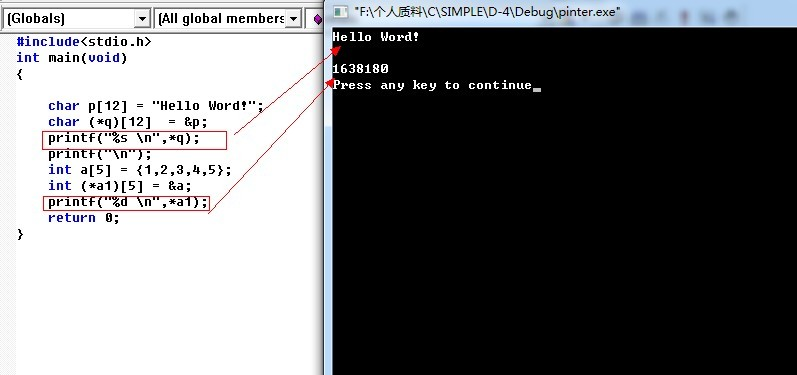0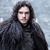printf(%s,p); printf会认为p是一个地址,指向一个字符串.

printf(%d,p) printf会认为p是一个值.而你的程序 *a1是一个地址,因此把地址当成值打印出来是没错的.

```#include<stdio.h>
int main(void)
{

char p = "Hello Word!";
char *q  = p;
printf("%s \n",q);
printf("\n");
int a = {1,2,3,4,5};
int *a1= a;
printf("%d \n",a1);
return 0;
}```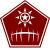0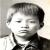q 是个指针空间，它里面存放的数据是指向一个地址，而该地址是一个空间，这个空间包含12个字符单元。

0
i

q 和 a1 都是数组指针，那么 *q 和 *a1 得到的应该都是数组，  如果数组充当函数变量的话，该数组就会被转换为指向数组第一个元素的指针，所以说两个 printf() 的参数都是两个数组第一个元素的地址。

```#include<stdio.h>
int main(void)
{

char p = "Hello Word!";
char (*q)  = &p;
char (*q1)  = &p;
printf("%s \n",*q);
printf("%p \n", q);
printf("%p \n", q1);
printf("\n");
int a = {1,2,3,4,5};
int (*a1) = &a;
int (*a2) = &a;
printf("%d \n",*a1);
printf("%p \n", a1);
printf("%p \n", a2);
return 0;
}```

0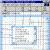(*a1)[] 是一个数组指针. 存放的内容都是指针. 所以 *a1 肯定是一个指针.

0
t

printf("%s\n", *q), 按照字符串打印, 字符串是以'\0'结尾, 而print("%d\n", *a1)是以整型打印, 即便q = &p, a1 = &a.

a1是存放地址的指针数组, 这个结果应该是0x01638180内存地址值

0
Y